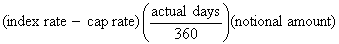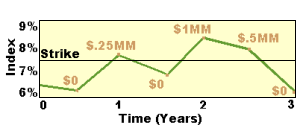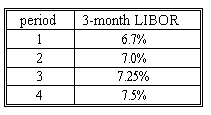### Why should I choose AnalystNotes?

AnalystNotes specializes in helping candidates pass. Period.

### Subject 2. Interest rate caps and floors

An interest-rate cap is an OTC derivative which protects the holder from rises in short-term interest rates by making a payment to the holder when an underlying interest rate (the index or reference interest rate) exceeds a specified strike rate (the cap rate). Caps are purchased for a premium, and typically have maturities between 1 and 7 years. They may make payments to the holder on a monthly, quarterly or semi-annual basis, with the period generally set equal to the maturity of the index interest rate.

Each period, the payment is determined by comparing the current level of the index interest rate with the cap rate. If the index rate exceeds the cap rate, the payment is based upon the difference between the two rates, the length of the period, and the contract's notional amount. Otherwise, no payment is made for that period. If a payment is due on a USD LIBOR cap, it is calculated asFor example, Exhibit A illustrates a 3-year, USD 200MM notional cap with 6-month LIBOR as its index rate, struck at 7.5%. The exhibit shows what the cap's payments would be under a hypothetical interest rate scenario.

Exhibit A: Cap PaymentsPayments made under a hypothetical interest rate scenario by a 3-year USD 200MM notional cap linked to 6-month USD LIBOR with strike rate of 7.5%. Values for the index rate are 6.25%, 7.75%, 7.00%, 8.50%, 8.00%, and 6.25%. These result in payments of USD 0MM, USD .25MM, USD 0MM, USD 1MM, USD .5MM, and USD 0MM.

Caps are frequently purchased by issuers of floating rate debt who wish to protect themselves from the increased financing costs that a rise in interest rates would entail.

An interest rate floor is just the opposite of an interest rate cap. If you want to read more, please see the appendix.

The buyer of an interest rate collar purchases a cap option to limit the maximum interest rate he will pay and sells a floor option to obtain a premium to pay for the cap. The effect of the combination is to confine interest rate payments to a range bounded by the strike prices of the cap and floor options. On the other side of the trade, an asset manager might sell a cap and give up the potential for unlimited upside yield in return for the assurance of a minimum return obtained by using the proceeds from the cap to purchase a floor.

As with a swap, a complex contract can be seen to be a package of basic contract. An interest rate cap can be seen as a series of European call options, or "caplets" on a specified reference rate, usually LIBOR. For example, if an interest rate cap is for three years and payments are made quarterly, this is equivalent to 12 interest rate options. Each option in the package is called a caplet.

Similarly, an interest rate floor can be seen as a series of European put options, or "floorlets".

Appendix: Interest Rate Floor

An interest rate floor is an OTC derivative which protects the holder from declines in short-term interest rates by making a payment to the holder when an underlying interest rate (the index or reference interest rate) falls below a specified strike rate (the floor rate). Floors are purchased for a premium, and typically have maturities between 1 and 7 years. They may make payments to the holder on a monthly, quarterly or semi-annual basis, with the period generally set equal to the maturity of the index interest rate.

Each period, the payment is determined by comparing the current level of the index interest rate with the floor rate. If the index rate is below the floor rate, the payment is based upon the difference between the two rates, the length of the period, and the contract's notional amount. Otherwise, no payment is made for that period. If a payment is due on a USD LIBOR floor, it is calculated asFor example, Exhibit B illustrates a 3-year, USD 200MM notional floor with 6-month LIBOR as its index rate, struck at 5.5%. The exhibit shows what the floor's payments would be under a hypothetical interest rate scenario.

Exhibit B: Floor PaymentsPayments made under a hypothetical interest rate scenario by a 3-year USD 200MM notional floor linked to 6-month USD LIBOR with strike rate of 5.5%. Values for the index rate are 6.75%, 5.25%, 6.25%, 4.50%, 5.00%, 6.75%. These result in payments of USD 0MM, USD .25MM, USD 0MM, USD 1MM, USD .5MM, and USD 0MM.

Floors might be used by purchasers of floating rate debt who wishes to protect themselves from the increased financing costs that a decline in interest rates would entail.

#### Practice Question 1

For a call option on a fixed income instrument, when interest rates increase:

I. Call option value increases.
II. Call option value decreases.
III. Fixed-income instrument's price increases.
IV. Fixed-income instrument's price decreases.

The value of a fixed income instrument changes inversely with interest rates.

#### Practice Question 2

When interest rates decrease, the value of short cap

A. increases.
B. decreases.

#### Practice Question 3

I. a package of calls on a fixed-income instrument.
II. a package of puts on a fixed-income instrument.
III. a package of call options on an interest rate.
IV. a package of put options on an interest rate.

#### Practice Question 4

Suppose that a 4-year cap has a cap rate of 7% and a notional amount of \$100 million. The frequency of settlement is quarterly and the reference rate is 3-month LIBOR. Assume that 3-month LIBOR for the next four quarters is as shown below. What is the payoff of the third quarter?There is no payoff to the cap is the cap rate exceeds 3-month LIBOR. For period 3, the payoff is determined by \$100 million x (3-month LIBOR - cap rate)/4 = \$62,500.

#### Practice Question 5

A caplet is like

A. a European call option on an interest rate.
B. a package of call options on an interest rate.
C. a series of interest rate caps.

Since a cap is equivalent to a package of interest rate options, each interest rate option in the package is called a caplet.

#### Practice Question 6

The strike rate of an interest cap is the

A. cap rate.
B. reference rate.
C. swap rate.

The predetermined level is called the strike rate. For a cap it is called the cap rate, and for a floor it is called the floor rate.

#### Practice Question 7

For a put option on a fixed income instrument, when interest rates increase:

I. Put option value will increase.
II. Put option value will decrease.
III. Fixed-income instrument's price will increase.
IV. Fixed-income instrument's price will decrease.

A. I and III
B. I and IV
C. II and IV

The value of a fixed income instrument changes inversely with interest rates.

#### Practice Question 8

I. a package of calls on a fixed-income instrument.
II. a package of puts on a fixed-income instrument.
III. a package of call options on an interest rate.
IV. a package of put options on an interest rate.

A. I and III
B. I and IV
C. II and IV

null

#### Practice Question 9

Suppose that a 4-year cap has a cap rate of 7% and a notional amount of \$100 million. The frequency of settlement is quarterly and the reference rate is 3-month LIBOR. Assume that 3-month LIBOR for the next four quarters is as shown below. What is the payoff of the third quarter?A. \$0.
B. \$250,000.
C. \$62,500.

There is no payoff to the cap as the cap rate exceeds 3-month LIBOR. For period 3, the payoff is determined by \$100 million x (3-month LIBOR - cap rate)/4 = \$62,500.

#### Practice Question 10

Suppose that a 4-year cap has a cap rate of 7% and a notional amount of \$100 million. The frequency of settlement is quarterly and the reference rate is 3-month LIBOR. Assume that 3-month LIBOR for the next four quarters is as shown below. What is the payoff of the third quarter?A. \$0.
B. \$250,000.
C. \$62,500.

There is no payoff to the cap if the cap rate exceeds 3-month LIBOR. For period 1 and 2 there is no payoff. For period 3, the payoff is (100m * 0.25%)/4 = 62,500.

#### Practice Question 11

A floorlet is equivalent to

A. a European put option on an interest rate.
B. a package of put options on an interest rate.
C. a series of interest rate floors.

Since a floor is equivalent to a package of interest rate options, each interest rate option in the package is called a floorlet.

#### Practice Question 12

If an interest rate cap is for three years and makes semi-annual payments, it can be viewed as

A. 6 caplets only.
B. 6 caplets, or 6 interest rate options.
C. 6 floorlets only.

Since a cap is equivalent to a package of interest rate options, each interest rate option in the package is called a caplet.

#### Practice Question 13

Which of the following combinations of interest rate caps and interest rate floors would replicate a plain vanilla swap in which a fixed rate of 6% would be payable in exchange for LIBOR?

A. Short interest rate caps and short interest rate floors, with both having the same exercise price and the same premium.
B. Long interest rate floors and short interest rate caps, with both having the same exercise price and the same premium.
C. Long interest rate caps and short interest rate floors, with both having the same exercise price and the same premium.

The long interest rate caps implies that if the LIBOR rate exceeds 6%, the cap holder would receive a payment, just as in the case of swap agreement. Similarly, a short interest rate floor would imply that if LIBOT drops below 6%, the seller will have to make a net payment equal to the difference between the prevailing LIBOR rate and 6%, just as in the case with the swap agreement.

#### Practice Question 14

Which of the following is most likely correct?

A. An interest rate floor can be defined as a series of interest rate call options with different expiry dates but same exercise rate.
B. An interest rate collar can be defined as a series interest rate puts (with different expiry dates but same exercise rate) and calls (also with different expiry dates but same exercise rate). Each put having the same expiry date as a call and vice versa.
C. An interest rate cap offers protection to the buyer (lender) if interest rates rise.